# Curves: Interpolated and Control Points

There are two fundamental ways to create free-form curves in Dynamo: specifying a collection of Points and having Dynamo interpolate a smooth curve between them, or a more low-level method by specifying the underlying control points of a curve of a certain degree. Interpolated curves are useful when a designer knows exactly the form a line should take, or if the design has specific constraints for where the curve can and cannot pass through. Curves specified via control points are in essence a series of straight line segments which an algorithm smooths into a final curve form. Specifying a curve via control points can be useful for explorations of curve forms with varying degrees of smoothing, or when a smooth continuity between curve segments is required.

To create an interpolated curve, simply pass in a collection of Points to the NurbsCurve.ByPoints method.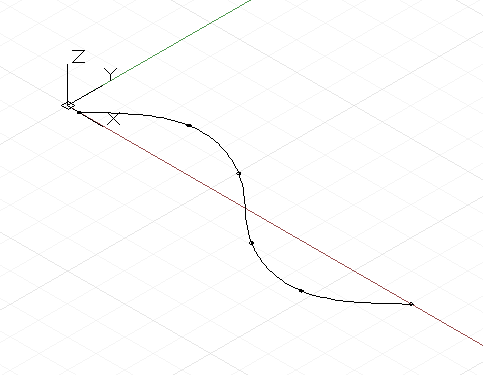``````num_pts = 6;

s = Math.Sin(0..360..#num_pts) * 4;

pts = Point.ByCoordinates(1..30..#num_pts, s, 0);

int_curve = NurbsCurve.ByPoints(pts);
``````

The generated curve intersects each of the input points, beginning and ending at the first and last point in the collection, respectively. An optional periodic parameter can be used to create a periodic curve which is closed. Dynamo will automatically fill in the missing segment, so a duplicate end point (identical to the start point) isn’t needed.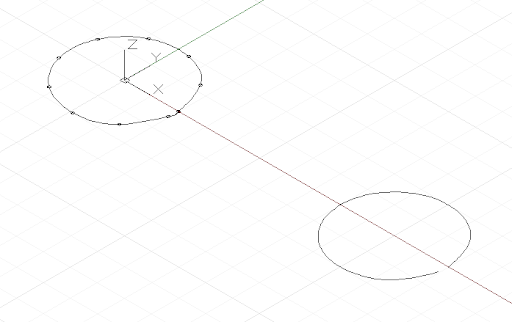``````pts = Point.ByCoordinates(Math.Cos(0..350..#10),
Math.Sin(0..350..#10), 0);

// create an closed curve
crv = NurbsCurve.ByPoints(pts, true);

// the same curve, if left open:
crv2 = NurbsCurve.ByPoints(pts.Translate(5, 0, 0),
false);
``````

NurbsCurves are generated in much the same way, with input points represent the endpoints of a straight line segment, and a second parameter specifying the amount and type of smoothing the curve undergoes, called the degree.* A curve with degree 1 has no smoothing; it is a polyline.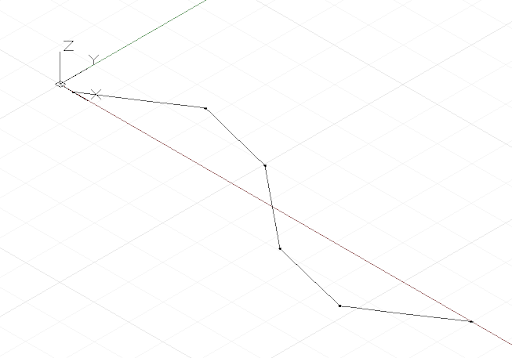``````num_pts = 6;

pts = Point.ByCoordinates(1..30..#num_pts,
Math.Sin(0..360..#num_pts) * 4, 0);

// a B-Spline curve with degree 1 is a polyline
ctrl_curve = NurbsCurve.ByControlPoints(pts, 1);
``````

A curve with degree 2 is smoothed such that the curve intersects and is tangent to the midpoint of the polyline segments: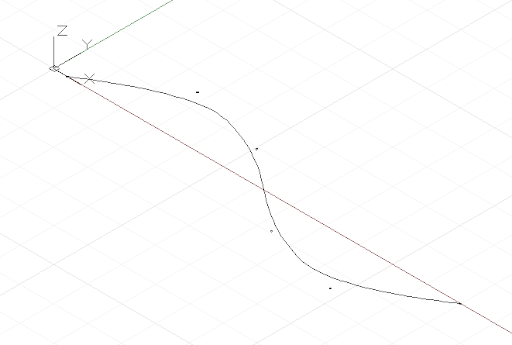``````num_pts = 6;

pts = Point.ByCoordinates(1..30..#num_pts,
Math.Sin(0..360..#num_pts) * 4, 0);

// a B-Spline curve with degree 2 is smooth
ctrl_curve = NurbsCurve.ByControlPoints(pts, 2);
``````

Dynamo supports NURBS (Non-uniform rational B-spline) curves up to degree 20, and the following script illustrates the effect increasing levels of smoothing has on the shape of a curve: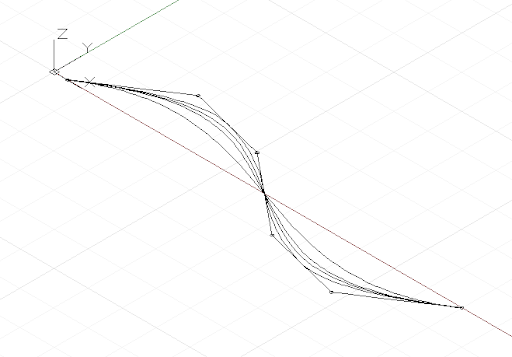``````num_pts = 6;

pts = Point.ByCoordinates(1..30..#num_pts,
Math.Sin(0..360..#num_pts) * 4, 0);

def create_curve(pts : Point[], degree : int)
{
return = NurbsCurve.ByControlPoints(pts,
degree);
}

ctrl_crvs = create_curve(pts, 1..11);
``````

Note that you must have at least one more control point than the degree of the curve.

Another benefit of constructing curves by control vertices is the ability to maintain tangency between individual curve segments. This is done by extracting the direction between the last two control points, and continuing this direction with the first two control points of the following curve. The following example creates two separate NURBS curves which are nevertheless as smooth as one curve: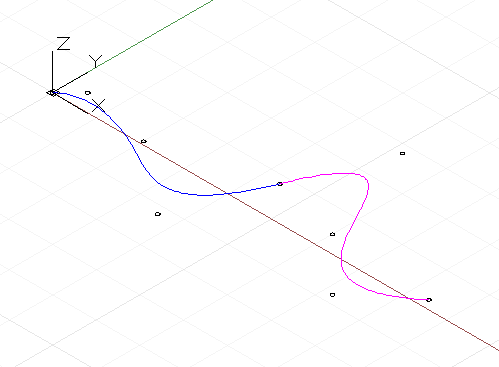``````pts_1 = {};

pts_1 = Point.ByCoordinates(0, 0, 0);
pts_1 = Point.ByCoordinates(1, 1, 0);
pts_1 = Point.ByCoordinates(5, 0.2, 0);
pts_1 = Point.ByCoordinates(9, -3, 0);
pts_1 = Point.ByCoordinates(11, 2, 0);

crv_1 = NurbsCurve.ByControlPoints(pts_1, 3);

pts_2 = {};

pts_2 = pts_1;
end_dir = pts_1.Subtract(pts_1.AsVector());

pts_2 = Point.ByCoordinates(pts_2.X + end_dir.X,
pts_2.Y + end_dir.Y, pts_2.Z + end_dir.Z);

pts_2 = Point.ByCoordinates(15, 1, 0);
pts_2 = Point.ByCoordinates(18, -2, 0);
pts_2 = Point.ByCoordinates(21, 0.5, 0);

crv_2 = NurbsCurve.ByControlPoints(pts_2, 3);
``````
• This is a very simplified description of NURBS curve geometry, for a more accurate and detailed discussion see Pottmann, et al, 2007, in the references.